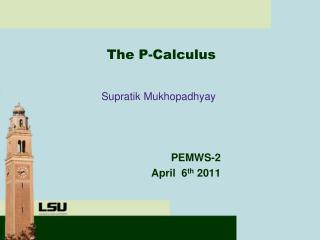# The P-Calculus - PowerPoint PPT PresentationDownload PresentationThe P-Calculus

The P-Calculus
Download Presentation## The P-Calculus

- - - - - - - - - - - - - - - - - - - - - - - - - - - E N D - - - - - - - - - - - - - - - - - - - - - - - - - - -
##### Presentation Transcript

1. The P-Calculus Supratik Mukhopadhyay PEMWS-2 April 6th 2011

2. Introduction & Motivation • Next generation data-intensive exascale computing architectures will have significantly different execution models: • Instead of data moving to work, work should move to data • Execution should be message-driven • Need to expose different granularities of parallelism: determinism, non-determinism, dataflow, globally asynchronous locally synchronous (GALS) semantics, … • Security & Reliability • Fault-tolerance

3. Motivation (Contd.) • Communicating Sequential Processes (CSP: Hoare 1978) served as the foundation for MPI • Limitations of CSP limit existing models with respect to expressiveness in terms of fine granularities of parallelism, fault-tolerance, security, localities, hierarchical partitioning of address spaces, … • Next generation ubiquitous high performance computing needs new execution models • What is the CSP for next generation exascale computing? • Abstract formal specification of parallel execution

4. Existing Specification Formalisms • Petri Nets • -calculus, CSP, CCS, Pi Calculus, Ambient Calculus, Actors, Kahn Process Networks, … • Do not support globally asynchronous locally synchronous semantics • Not message driven • Cannot expose different granularities of parallelism (deterministic/dataflow, nondeterministic, fine-grained, coarse-grained) • Does not support work moving to data

5. The P-calculus: A Specifcation Formalism for Exascale Computation • Nominal process calculus as a formal execution model for future-generation exascale computation • Has formal operational semantics involving interactions of external and internal actions • Allows answering questions about the execution models unambigously • Allows (automatic) checking for inconsistencies and incompleteness in the execution model • Allows checking (automatically) whether a claimed implementation of an execution model such as ParalleX or Codelets really implements it

6. The P-calculus (contd.) • Uniform representation of synchronous and asynchronous semantics • Has an equational theory that allows comparison of different implementations of an execution model • Equipped with a type system (the first order P-calculus) for correctness, security, …

7. The Untyped P-calculus • Chromosomes: Primitive computing elements in the P-calculus • Internal Actions • External Actions • Chromatins • Regulators • Boards • Nucleus

8. Chromosomes • Chromosomes are the most basic kind of computational entity defined in the P-Calculus. • Their role is to execute fundamental computations.

9. Chromosomes (Contd.) • Chromosomes may also declare methods: • The set of declared methods is partitioned into private and public methods. Formal Parameters

10. Internal Actions • A chromosome may perform either an internal or external action.

11. Chromatins • Chromosomes are only capable of performing sequential computations. • A Chromatin is defined as a group of coexisting chromosomes organized together by parallel composition.

12. Regulators • Aside from being executed computations need to be managed. • Managing computations involves: • Controlling and synchronizing data access • Moving work to data AS1 @T(x) y (y)… @C(y)

13. Regulators – Guarded Commands • Each regulator is denoted by a deterministic state machine that is defined by a set of guarded commands. • Each guard is denoted by an event which triggers an action. At most one of the guards can be triggered by an event

14. Regulators – Event Language • Each event can be an atomic event (extending Parnas). • Or an event could also be a complex event. • An action (for now) is the creation of a chromosome.

15. Board • Regulators can coexist may be composed • A “parallel” composition of regulators is known as a board. AS2 @T(x) @C(y) @F(z)

16. Nucleus • We can perform computations (via chromatins and chromosomes) • We can manage computations (via boards and regulators). • A nucleus is a composition of coexistingchromatins and boards. AS2 @T(x) @C(y) @F(z) Nucleus

17. Activity Space • Computations need a context i.e. a place in which they take place. • An Activity Space is the context in which a cooperating and coexisting chromatin and a board interacts (i.e., a nucleus exists).

18. Example – Activity Spacesand Nuclei AS1 AS2 let x = … .let y = …. @T(x) @C(y) let z = … .<<z>> @F(z)

19. System • A System is a composition of Activity Spaces • Multilevel hierarchy

20. External Actions • Boards and chromatins were capable of performing and coordinating/managing internal computations. • External actions coordinate computations across Activity Spaces.

21. Example – External Actions AS1 out AS1.in AS2 AS2 <<foo>> let x = … .let y = …. In AS3 (foo) @T(x) @C(y) AS3 let z = … .<<z>> @F(z)

22. Operational Semantics – Visual Example let x = getInput() let z = shuffle(y) let y = sort(x)^f(sort,cont) (y^cont) <<z>>^cont^^’’ @C(cont) <<y>>^cont’^^’ ’

23. Example – Operational Semantics

24. Example (Cont’d)

25. Future Work • First Order P-calculus • Confluence Properties • Tool Building • Query Languages

26. Questions?